# ML 106: Data Poisoning (10 pts extra)

## What You Need

• A Web browser

## Purpose

To investigate the effect of altering the training data on a machine learning system.

In a browser, go to

From the menu, click File, "New notebook".

## Preparing a Classification Model

Execute these commands to prepare the starting classification model we are using. This is the model that was created at the end of project ML 105.

It uses a training set of 2000 images and a testing set of 1000 images. Each image is a handwritten digit from 0 through 9, and the task is to identify the digit.

```from sklearn.datasets import fetch_openml from sklearn.svm import SVC import numpy # Fetch the original data mnist = fetch_openml('mnist_784', as_frame=False, parser="auto") print(mnist.DESCR) X, y = mnist.data, mnist.target # Prepare shorter training and testing sets X_train_orig = numpy.array([[]]).reshape(0, 784) y_train_orig = numpy.array([]) X_test_orig = numpy.array([]).reshape(0, 784) y_test_orig = numpy.array([]) for i in range(2000): X_train_orig = numpy.concatenate((X_train_orig, [X[i]]), axis=0) y_train_orig = numpy.append(y_train_orig, y[i]) for i in range(1000): X_test_orig = numpy.concatenate((X_test_orig, [X[i+ 60000]]), axis=0) y_test_orig = numpy.append(y_test_orig, y[i + 60000]) # Train the model svm_clf = SVC(random_state=42) svm_clf.fit(X_train_orig, y_train_orig) # Print results print("Correct\tPredicted") for i in range(5): print(y_test_orig[i], "\t", svm_clf.predict([X_test_orig[i]])) ```
You see the first five results, as shown below. All these predictions are correct.## Viewing Predictions

Execute these commands display a summary of the model's predictions.
```prediction_correct = [0,0,0,0,0,0,0,0,0,0] prediction_incorrect = [0,0,0,0,0,0,0,0,0,0] for i in range(1000): p = svm_clf.predict([X_test_orig[i]]) if p == y_test_orig[i]: prediction_correct[int(p)] += 1 else: prediction_incorrect[int(p)] += 1 print("Value\tCorrect\tIncorrect") for i in range(10): print(i, "\t", prediction_correct[i], "\t", prediction_incorrect[i]) ```
As shown below, the original model gets only 2 1's incorrect, and 12 2's incorrect.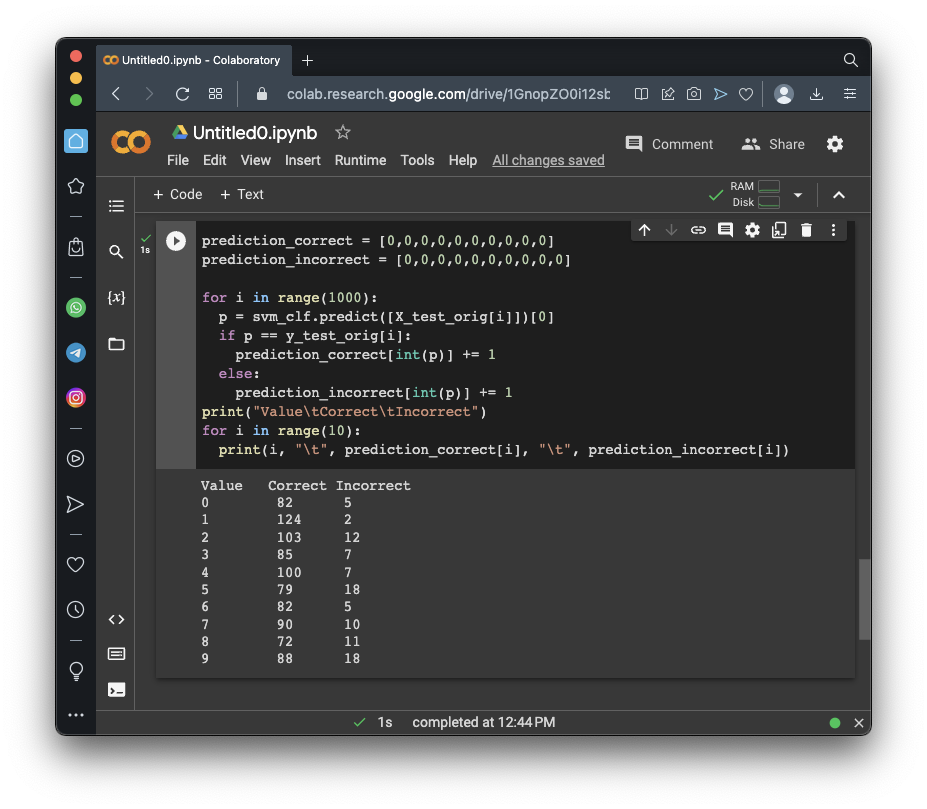## Summarizing the Training and Testing Sets

Execute these commands to summarize the training and testing sets.
```test_count = [0,0,0,0,0,0,0,0,0,0] train_count = [0,0,0,0,0,0,0,0,0,0] for yy in y_train_orig: train_count[int(yy)] += 1 for yy in y_test_orig: test_count[int(yy)] += 1 print("Correct\tTrain\tTest") for i in range(10): print(i, "\t", train_count[i], "\t", test_count[i]) ```
As shown below, the training set contains approximlately 200 of each The test set contains approximately 100 of each digit.

Notice that the training set contains 220 1's and 198 2's.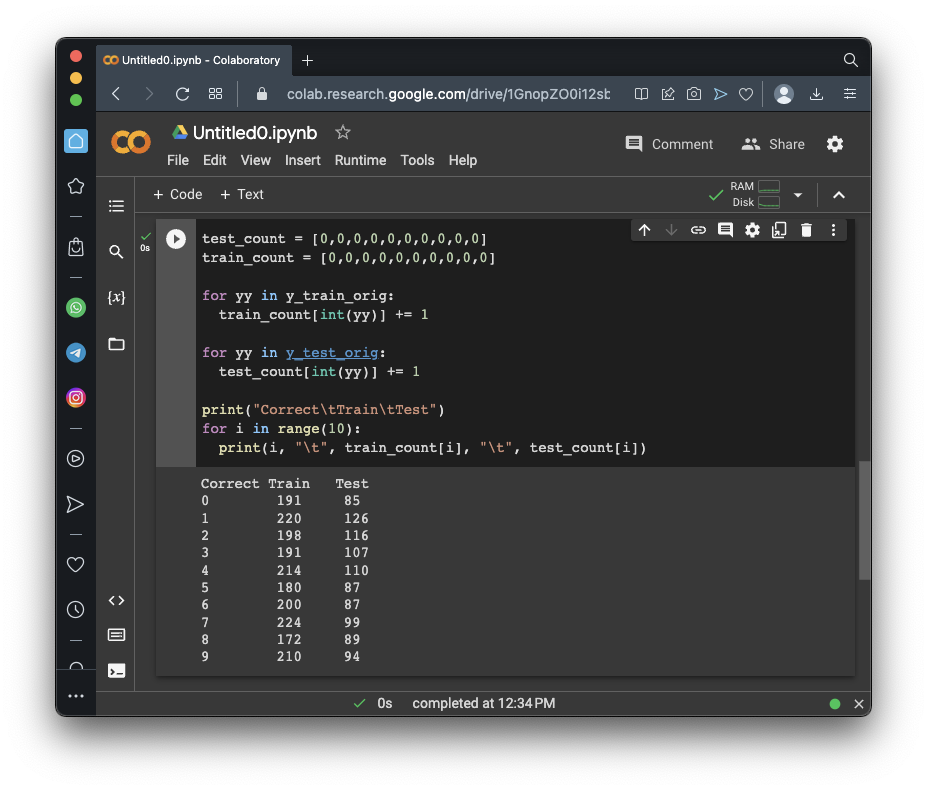## Poisoning 10 of the 1's

We're going to trick the model into identifying more 1's as 2's.

Execute these commands to prepare a y_train_poisoned list with the first 10 1's changed to 2's:

```y_train_poisoned = y_train_orig.copy() count_before = [0,0,0,0,0,0,0,0,0,0] count_after = [0,0,0,0,0,0,0,0,0,0] for yi in y_train_poisoned: count_before[int(yi)] += 1 number_poisoned = 0 for i in range(2000): if y_train_poisoned[i] == '1': y_train_poisoned[i] = '2' number_poisoned += 1 if number_poisoned == 10: break for yi in y_train_poisoned: count_after[int(yi)] += 1 print("Correct\tBefore\tAfter") for i in range(10): print(i, "\t", count_before[i], "\t", count_after[i]) ```
As shown below, poisoning caused the number of 1's to fall by 10 and the number of 2's to rise by 10.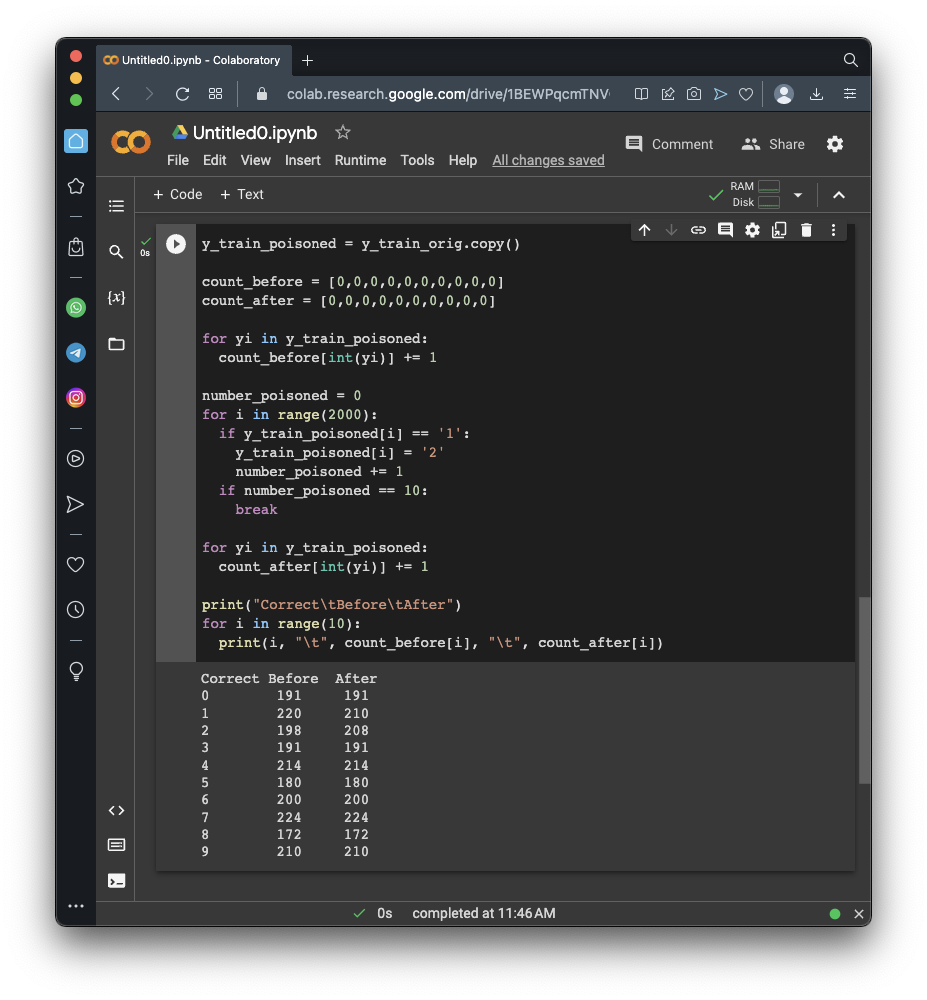## Training from the Poisoned Data

Execute these commands to create and train a model on the poisoned data, and display its predictions.
```svm_clf_poisoned = SVC(random_state=42) svm_clf_poisoned.fit(X_train_orig, y_train_poisoned) prediction_correct = [0,0,0,0,0,0,0,0,0,0] prediction_incorrect = [0,0,0,0,0,0,0,0,0,0] for i in range(1000): p = svm_clf_poisoned.predict([X_test_orig[i]]) if p == y_test_orig[i]: prediction_correct[int(p)] += 1 else: prediction_incorrect[int(p)] += 1 print("Value\tCorrect\tIncorrect") for i in range(10): print(i, "\t", prediction_correct[i], "\t", prediction_incorrect[i]) ```
As shown below, the poisoned model gets only 2 1's incorrect, and 11 2's incorrect. The poisoning had very little effect, and even made the prediction slightly better for 2's!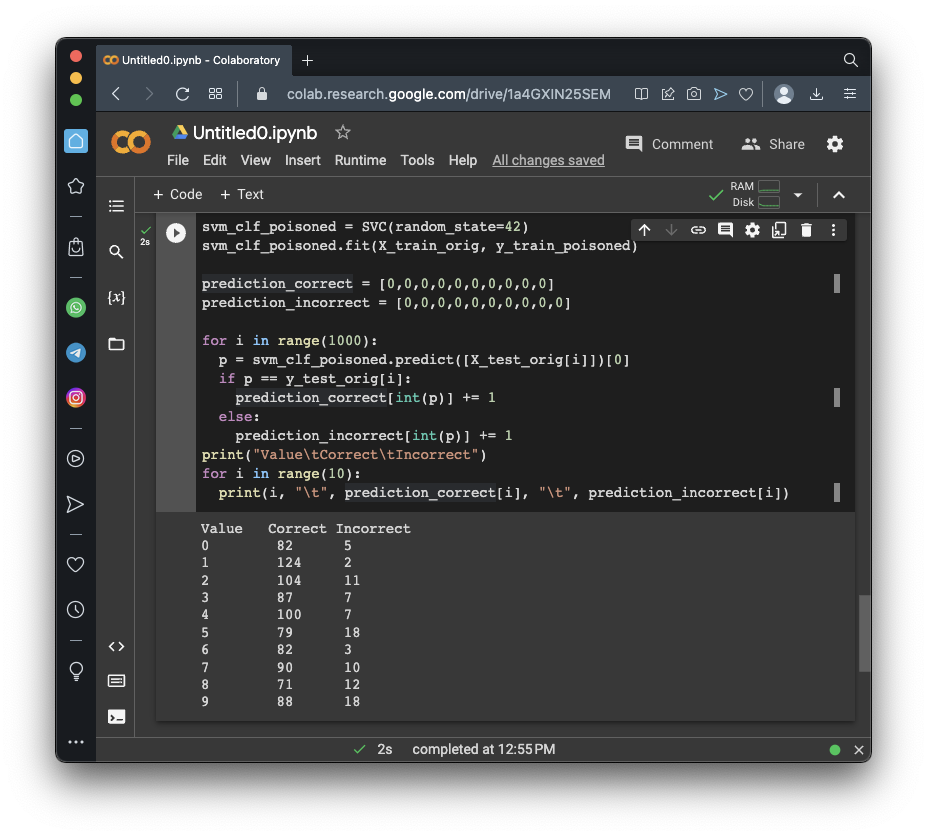## Poisoning 30 of the 1's

Execute these commands to prepare a y_train_poisoned list with the first 30 1's changed to 2's:
```y_train_poisoned = y_train_orig.copy() count_before = [0,0,0,0,0,0,0,0,0,0] count_after = [0,0,0,0,0,0,0,0,0,0] for yi in y_train_poisoned: count_before[int(yi)] += 1 number_poisoned = 0 for i in range(2000): if y_train_poisoned[i] == '1': y_train_poisoned[i] = '2' number_poisoned += 1 if number_poisoned == 30: break for yi in y_train_poisoned: count_after[int(yi)] += 1 print("Correct\tBefore\tAfter") for i in range(10): print(i, "\t", count_before[i], "\t", count_after[i]) ```
As shown below, poisoning caused the number of 1's to fall by 30 and the number of 2's to rise by 30.## Training from the Poisoned Data

Execute these commands to create and train a model on the poisoned data, and display its predictions.
```svm_clf_poisoned = SVC(random_state=42) svm_clf_poisoned.fit(X_train_orig, y_train_poisoned) prediction_correct = [0,0,0,0,0,0,0,0,0,0] prediction_incorrect = [0,0,0,0,0,0,0,0,0,0] for i in range(1000): p = svm_clf_poisoned.predict([X_test_orig[i]]) if p == y_test_orig[i]: prediction_correct[int(p)] += 1 else: prediction_incorrect[int(p)] += 1 print("Value\tCorrect\tIncorrect") for i in range(10): print(i, "\t", prediction_correct[i], "\t", prediction_incorrect[i]) ```
As shown below, the poisoned model gets only 2 1's incorrect, and 13 2's incorrect. The poisoning still had very little effect.## Flag ML 106.1: Poisoning 100 (10 pts)

Poison 100 of the 1's to mislabel them as 2's.

The flag is the number of incorrect 2's, covered by a green rectangle in the image below.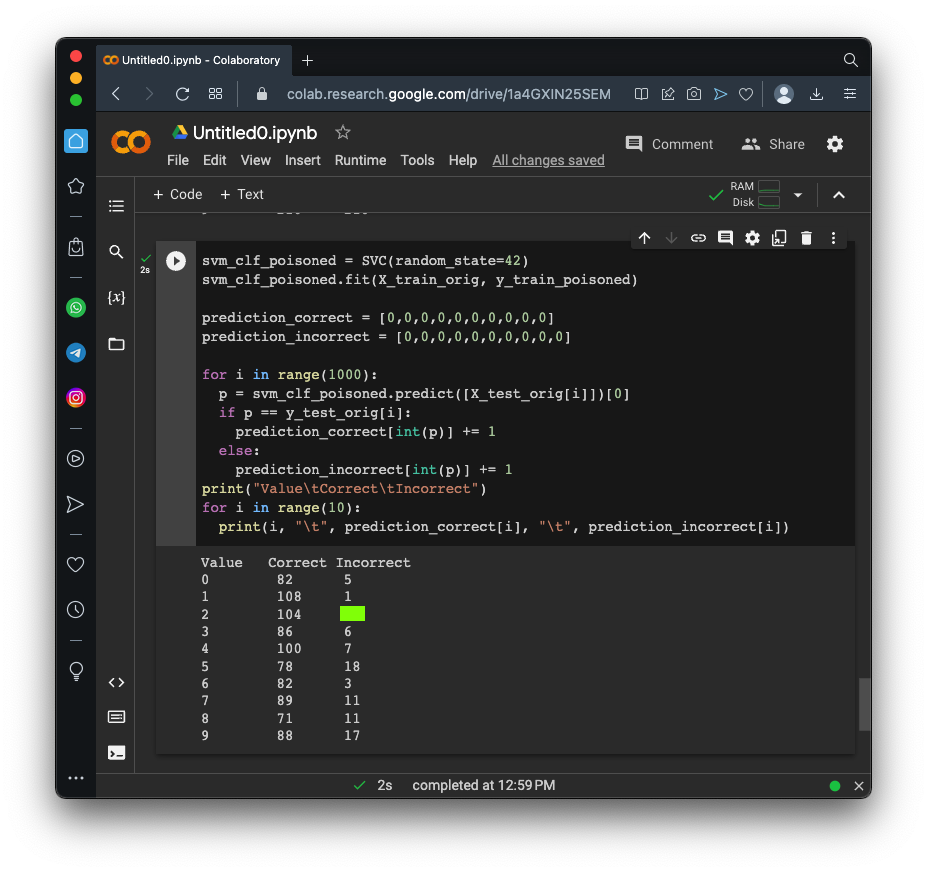## Sources

Hands-On Machine Learning with Scikit-Learn, Keras, and TensorFlow 3rd Edition, Kindle Edition
Top 5 Security Threats Facing Artificial Intelligence and Machine Learning

Posted 4-26-23